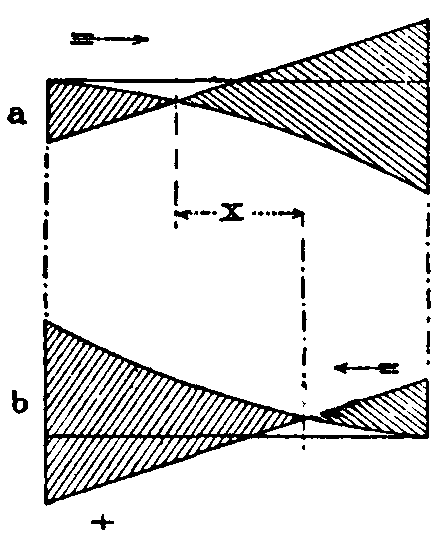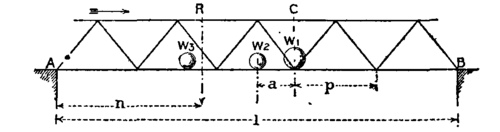H = H = M/h

and the intensity of stress of tension or compression is

f = M/Ah,

f = M/Ah.

If A is the area of the plate web in a vertical section, the intensity of shearing stress is

f = S/A

and the intensity on horizontal sections is the same. If the web is a braced web, then the vertical component of the stress in the web bars cut by the section must be equal to S.Fig. 38.

21. Method of Sections. A. Ritter's Method. - In the case of braced structures the following method is convenient: When a section of a girder can be taken cutting only three bars, the stresses in the bars can be found by taking moments. In fig. 38 m n cuts three bars, and the forces in the three bars cut by the section are C, S and T. There are to the left of the section the external forces, R, W, W. Let s be the perpendicular from O, the join of C and T on the direction of S; t the perpendicular from A, the join of C and S on the direction of T; and c the perpendicular from B, the join of S and T on the direction of C. Taking moments about O,

R-W(x+a)-W(x+2a) = Ss;

R3a-W2a-Wa = Tt;

R2a-Wa = Cc

Or generally, if M M M are the moments of the external forces to the left of O, A, and B respectively, and s, t and c the perpendiculars from O, A and B on the directions of the forces cut by the section, then

Ss = M; Tt = M and Cc = M.

Still more generally if H is the stress on any bar, h the perpendicular distance from the join of the other two bars cut by the section, and M is the moment of the forces on one side of that join,

Hh = M.Fig. 40.Fig. 39.

22. Distribution of Bending Moment and Shearing Force. - Let a girder of span l, fig. 39, supported at the ends, carry a fixed load W at m from the right abutment. The reactions at the abutments are R = Wm/l and R = W(l-m)/l. The shears on vertical sections to the left and right of the load are R and -R, and the distribution of shearing force is given by two rectangles. Bending moment increases uniformly from either abutment to the load, at which the bending moment is M = Rm = R(l-m). The distribution of bending moment is given by the ordinates of a triangle. Next let the girder carry a uniform load w per ft. run (fig. 40). The total load is wl; the reactions at abutments, R = R = &FRAC12;wl. The distribution of shear on vertical sections is given by the ordinates of a sloping line. The greatest bending moment is at the centre and = M = ⅛wl2. At any point x from the abutment, the bending moment is M = &FRAC12;wx(l-x), an equation to a parabola.

23. Shear due to Travelling Loads. - Let a uniform train weighing w per ft. run advance over a girder of span 2c, from the left abutment. When it covers the girder to a distance x from the centre (fig. 41) the total load is w(c+x); the reaction at B is

 R = w(c+x)× c+x4c = w4c (c+x)&SUP2;,Fig. 42.Fig. 41.

which is also the shearing force at C for that position of the load. As the load travels, the shear at the head of the train will be given by the ordinates of a parabola having its vertex at A, and a maximum F = -&FRAC12;wl at B. If the load travels the reverse way, the shearing force at the head of the train is given by the ordinates of the dotted parabola. The greatest shear at C for any position of the load occurs when the head of the train is at C. For any load p between C and B will increase the reaction at B and therefore the shear at C by part of p, but at the same time will diminish the shear at C by the whole of p. The web of a girder must resist the maximum shear, and, with a travelling load like a railway train, this is greater for partial than for complete loading. Generally a girder supports both a dead and a live load. The distribution of total shear, due to a dead load w per ft. run and a travelling load w per ft. run, is shown in fig. 42, arranged so that the dead load shear is added to the maximum travelling load shear of the same sign.Fig. 43.

24. Counterbracing. - In the case of girders with braced webs, the tension bars of which are not adapted to resist a thrust, another circumstance due to the position of the live load must be considered. For a train advancing from the left, the travelling load shear in the left half of the span is of a different sign from that due to the dead load. Fig. 43 shows the maximum shear at vertical sections due to a dead and travelling load, the latter advancing (fig. 43, a) from the left and (fig. 43, b) from the right abutment. Comparing the figures it will be seen that over a distance x near the middle of the girder the shear changes sign, according as the load advances from the left or the right. The bracing bars, therefore, for this part of the girder must be adapted to resist either tension or thrust. Further, the range of stress to which they are subjected is the sum of the stresses due to the load advancing from the left or the right.Fig. 46.